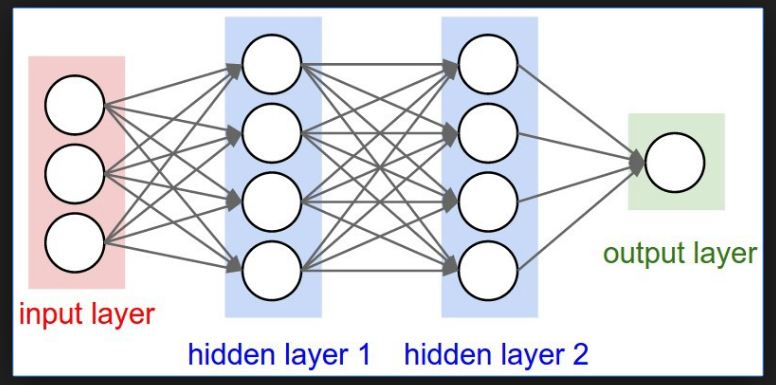/  Hidden Layers   /  Hidden Layers in Neural Networks## What Are Hidden Layers?

The Hidden Layers is the important topic to understand when we are working with Machine Learning models. Particularly in this topic we concentrate on the Hidden Layers of a neural network layer.

## Neural Network Layers:

The layer is a group, where number of neurons together and the layer is used for the holding a collection of neurons. Simply we can say that the layer is a container of neurons. In these layers there will always be an input and output layers and we have zero or more number of hidden layers. The entire learning process of neural network is done with layers. In this the neurons are placed within the layer and that each layer has its purpose and each neuron perform the same function. These are used to calculate the weighted sum of inputs and weights and add the bias and execute the required activation function.

Let’s see the different types of layers in neural networks.

## Input Layer:

The input layer is the most responsible layer for receiving the inputs and these inputs are loaded from some external sources like csv file or web service etc.. In neural networks we must maintain one input layer to takes the inputs and perform some calculations through its neurons and then the output is transmitted to the next layers. Simply input layer takes the inputs and output layers produce the final output results.

In this the number of neurons in an input layer depends on the shape of our training data. And having the node to capture the bias term.

## Output Layer:

The output layer is mostly responsible for producing the final output results. There must be always an output layer in the neural networks. The output layer takes the inputs which are passed in from the layers before it, and performs the calculations through its neurons and then the output is computed.

But in any complex neural networks the output layer receives inputs from the previous hidden layers. The output is a regressor then the output layer has a single node. And it is classifier it is also having the single node and if you use a probabilistic Activation function such as SoftMax then the output layer has one node per one class label of our model.

## HiddenLayer :

The Hidden layers make the neural networks as superior to machine learning algorithms. The hidden layers are placed in between the input and output layers that’s why these are called as hidden layers. And these hidden layers are not visible to the external systems and these are private to the neural networks. There should be zero or more than zero hidden layers in the neural networks. For large majority of problems one hidden layer is sufficient.

Basically, each hidden layer contains same number of  neurons and large number of hidden layers in neural network the longer it will take for the neural network produce the output  and if any complex problems by using the hidden layers the neural networks can solve.

Experiments have shown us that the optimum number of neurons in a hidden layer can be determined by:The factor is used to prevent over-fitting and it is a number between 1–10.

To understand what activation functions are go through this link ,

https://www.i2tutorials.com/technology/activation-functions-in-deep-learning/